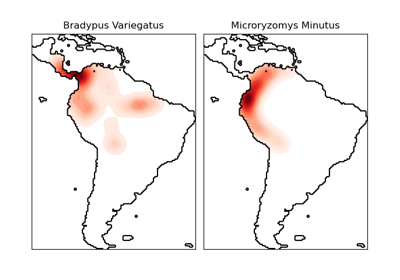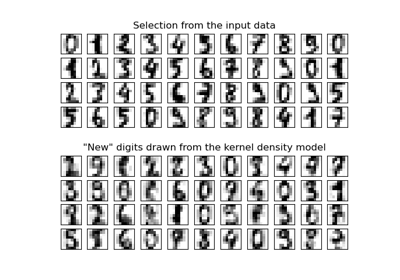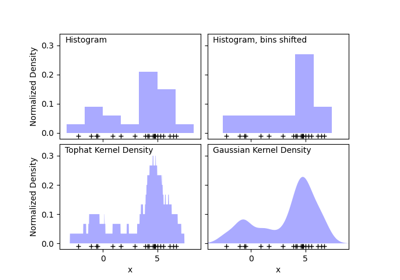# sklearn.neighbors.KernelDensity¶

class sklearn.neighbors.KernelDensity(*, bandwidth=1.0, algorithm='auto', kernel='gaussian', metric='euclidean', atol=0, rtol=0, breadth_first=True, leaf_size=40, metric_params=None)[source]

Kernel Density Estimation.

Read more in the User Guide.

Parameters:
bandwidthfloat, default=1.0

The bandwidth of the kernel.

algorithm{‘kd_tree’, ‘ball_tree’, ‘auto’}, default=’auto’

The tree algorithm to use.

kernel{‘gaussian’, ‘tophat’, ‘epanechnikov’, ‘exponential’, ‘linear’, ‘cosine’}, default=’gaussian’

The kernel to use.

metricstr, default=’euclidean’

Metric to use for distance computation. See the documentation of scipy.spatial.distance and the metrics listed in distance_metrics for valid metric values.

Not all metrics are valid with all algorithms: refer to the documentation of BallTree and KDTree. Note that the normalization of the density output is correct only for the Euclidean distance metric.

atolfloat, default=0

The desired absolute tolerance of the result. A larger tolerance will generally lead to faster execution.

rtolfloat, default=0

The desired relative tolerance of the result. A larger tolerance will generally lead to faster execution.

If true (default), use a breadth-first approach to the problem. Otherwise use a depth-first approach.

leaf_sizeint, default=40

Specify the leaf size of the underlying tree. See BallTree or KDTree for details.

metric_paramsdict, default=None

Additional parameters to be passed to the tree for use with the metric. For more information, see the documentation of BallTree or KDTree.

Attributes:
n_features_in_int

Number of features seen during fit.

New in version 0.24.

tree_BinaryTree instance

The tree algorithm for fast generalized N-point problems.

feature_names_in_ndarray of shape (n_features_in_,)

Names of features seen during fit. Defined only when X has feature names that are all strings.

New in version 1.0.

sklearn.neighbors.KDTree

K-dimensional tree for fast generalized N-point problems.

sklearn.neighbors.BallTree

Ball tree for fast generalized N-point problems.

Examples

Compute a gaussian kernel density estimate with a fixed bandwidth.

>>> from sklearn.neighbors import KernelDensity
>>> import numpy as np
>>> rng = np.random.RandomState(42)
>>> X = rng.random_sample((100, 3))
>>> kde = KernelDensity(kernel='gaussian', bandwidth=0.5).fit(X)
>>> log_density = kde.score_samples(X[:3])
>>> log_density
array([-1.52955942, -1.51462041, -1.60244657])


Methods

 fit(X[, y, sample_weight]) Fit the Kernel Density model on the data. get_params([deep]) Get parameters for this estimator. sample([n_samples, random_state]) Generate random samples from the model. score(X[, y]) Compute the total log-likelihood under the model. Compute the log-likelihood of each sample under the model. set_params(**params) Set the parameters of this estimator.
fit(X, y=None, sample_weight=None)[source]

Fit the Kernel Density model on the data.

Parameters:
Xarray-like of shape (n_samples, n_features)

List of n_features-dimensional data points. Each row corresponds to a single data point.

yNone

Ignored. This parameter exists only for compatibility with Pipeline.

sample_weightarray-like of shape (n_samples,), default=None

List of sample weights attached to the data X.

New in version 0.20.

Returns:
selfobject

Returns the instance itself.

get_params(deep=True)[source]

Get parameters for this estimator.

Parameters:
deepbool, default=True

If True, will return the parameters for this estimator and contained subobjects that are estimators.

Returns:
paramsdict

Parameter names mapped to their values.

sample(n_samples=1, random_state=None)[source]

Generate random samples from the model.

Currently, this is implemented only for gaussian and tophat kernels.

Parameters:
n_samplesint, default=1

Number of samples to generate.

random_stateint, RandomState instance or None, default=None

Determines random number generation used to generate random samples. Pass an int for reproducible results across multiple function calls. See Glossary.

Returns:
Xarray-like of shape (n_samples, n_features)

List of samples.

score(X, y=None)[source]

Compute the total log-likelihood under the model.

Parameters:
Xarray-like of shape (n_samples, n_features)

List of n_features-dimensional data points. Each row corresponds to a single data point.

yNone

Ignored. This parameter exists only for compatibility with Pipeline.

Returns:
logprobfloat

Total log-likelihood of the data in X. This is normalized to be a probability density, so the value will be low for high-dimensional data.

score_samples(X)[source]

Compute the log-likelihood of each sample under the model.

Parameters:
Xarray-like of shape (n_samples, n_features)

An array of points to query. Last dimension should match dimension of training data (n_features).

Returns:
densityndarray of shape (n_samples,)

Log-likelihood of each sample in X. These are normalized to be probability densities, so values will be low for high-dimensional data.

set_params(**params)[source]

Set the parameters of this estimator.

The method works on simple estimators as well as on nested objects (such as Pipeline). The latter have parameters of the form <component>__<parameter> so that it’s possible to update each component of a nested object.

Parameters:
**paramsdict

Estimator parameters.

Returns:
selfestimator instance

Estimator instance.

## Examples using sklearn.neighbors.KernelDensity¶Kernel Density Estimate of Species Distributions

Kernel Density Estimate of Species DistributionsKernel Density Estimation

Kernel Density EstimationSimple 1D Kernel Density Estimation

Simple 1D Kernel Density Estimation